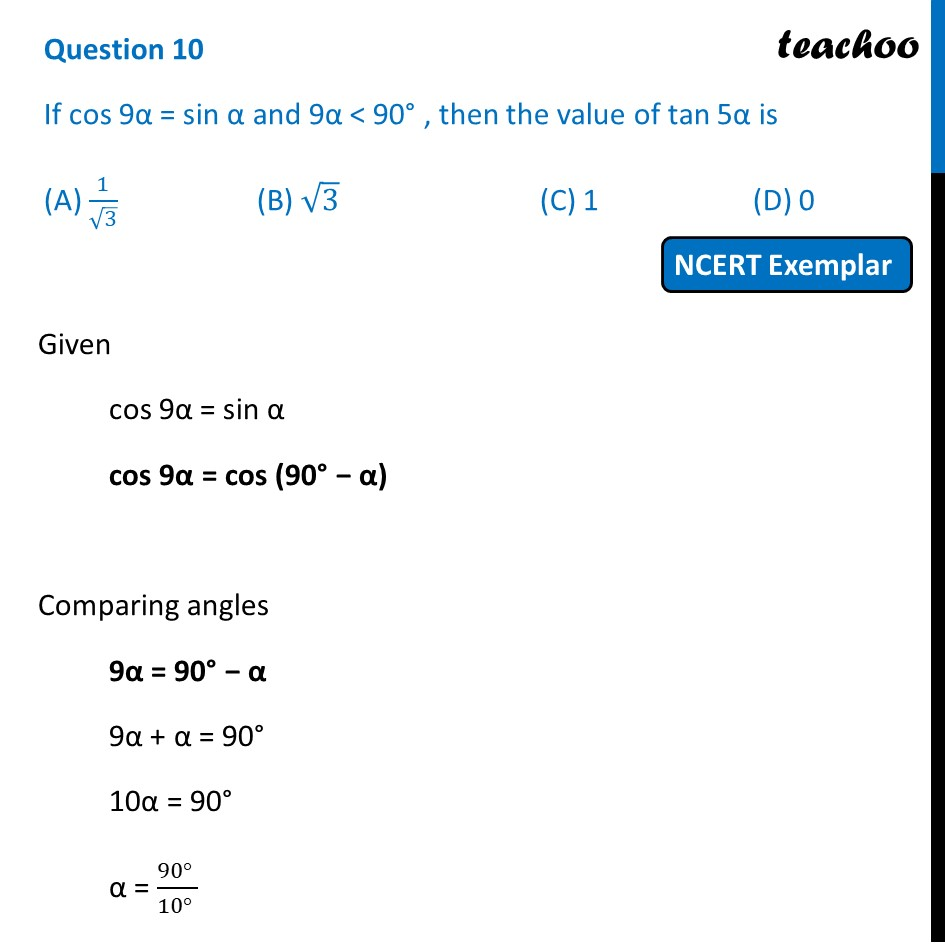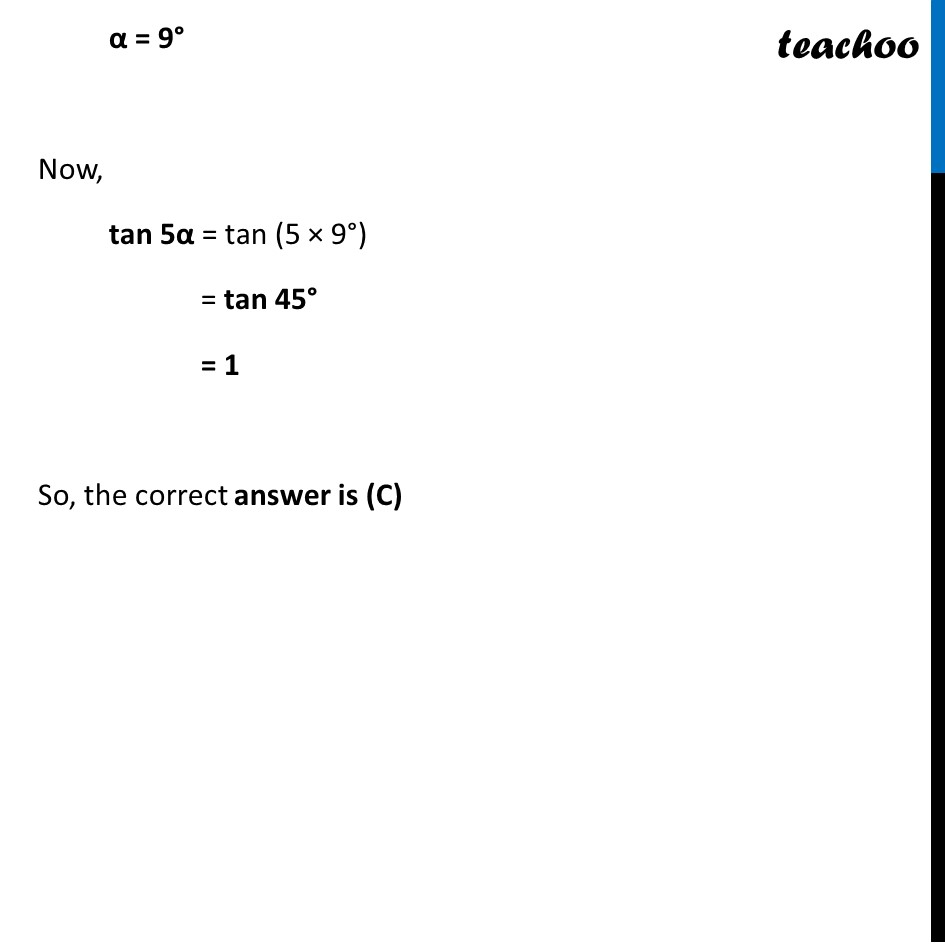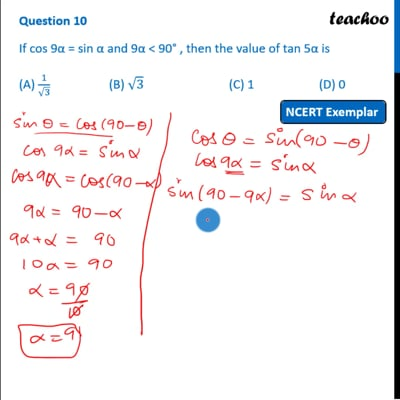NCERT Exemplar - MCQ

Chapter 8 Class 10 Introduction to Trignometry
Serial order wise

## (A) 1/√3   (B) √3   (C) 1  (D) 0This video is only available for Teachoo black users

Learn in your speed, with individual attention - Teachoo Maths 1-on-1 Class

### Transcript

Question 10 If cos 9α = sin α and 9α < 90° , then the value of tan 5α is (A) 1/√3 (B) √3 (C) 1 (D) 0 Given cos 9α = sin α cos 9α = cos (90° − α) Comparing angles 9α = 90° − α 9α + α = 90° 10α = 90° α = (90° )/(10° ) α = 9° Now, tan 5α = tan (5 × 9°) = tan 45° = 1 So, the correct answer is (C)